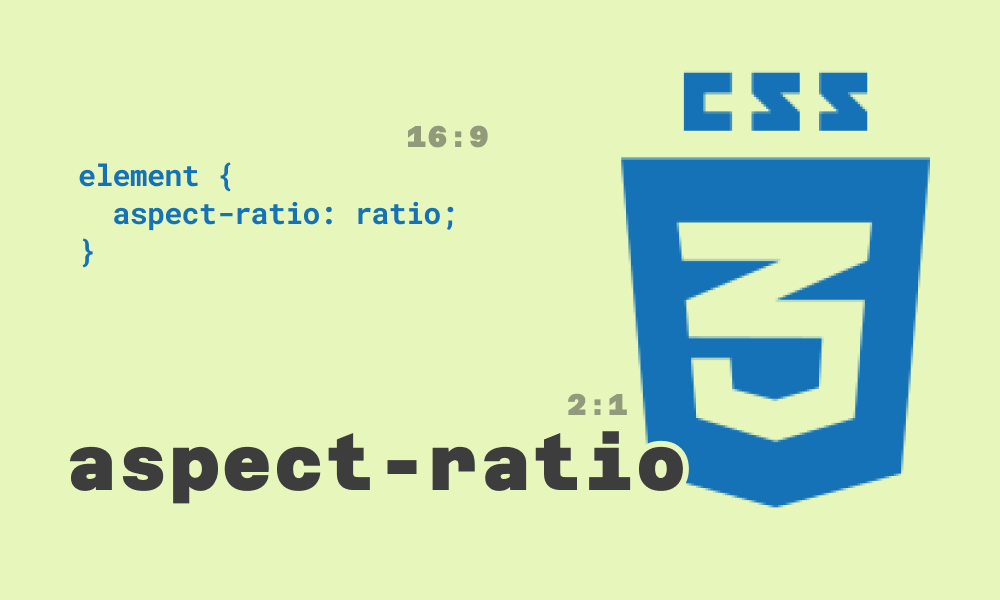# The Aspect Ratio Property in CSS

June 18th, 2022 | 2 mins read

#cssHere's a video if you'd prefer that: https://www.youtube.com/shorts/9h-zZ8FupUs

The `aspect-ratio` property is a new CSS property that allows you to set the aspect ratio of an element. It allows for the auto-sizing of elements based on the specified aspect ratio.

With aspect ratios, when you set the width of an element, the height of the element is automatically calculated to match the ratio.

The syntax for the `aspect-ratio` property is:

``````element {
aspect-ratio: width / height;
}``````

Here are some example ratios:

• a ratio of 1/2 would mean that the height of the element is twice the width of the element
• 16/9 would mean that the element's height is 9/16 times the element's width.
• 1 would mean that the element's height is the same as the element's width.

You can also use floating numbers. Instead of fraction 1/2, you can use 0.5.

Let's see some visual examples.

``````<div class="container">
<div class="box"></div>
</div>``````
``````.container {
width: 200px;
height: 200px;
border: 1px solid #0a0a22;
}

.box {
background-color: #0a0a22;
width: 100px;
aspect-ratio: 1;
}``````

An aspect ratio of 1 (or 1/1) means that the element's height is the same as the element's width. The result of the above is:

Another example:

``````.box {
background-color: #0a0a22;
width: 100px;
aspect-ratio: 1/2;}``````

An aspect ratio of 1/2 means that the element's height is twice the element's width. The result of the above is:

And another example with the height set:

``````.box {
background-color: #0a0a22;
height: 100px;
aspect-ratio: 9/16;}``````

With the height set, an aspect ratio of 9/16 means that the element's width is 9/16 times the element's height. The result of the above is:

## Without height or width set

What if you do not set a width or height? Let's see an example:

``````.box {
background-color: #0a0a22;
aspect-ratio: 1/2;
}``````

As you can see, without an aspect ratio, the box's width is 100%, and the height is 50% to match the ratio of 2/1. If you inverse the ratio to 1/2, the result is:

The width is 100%, and the height is two times the width to match the ratio of 1/2.

`aspect-ratio` is a very handy property that saves you the stress of setting the width and height of an element and changing them for different screen sizes for responsive layouts. You set an aspect ratio, and all you need to do is set either the width or the height (or even none), and CSS handles the calculation for you.